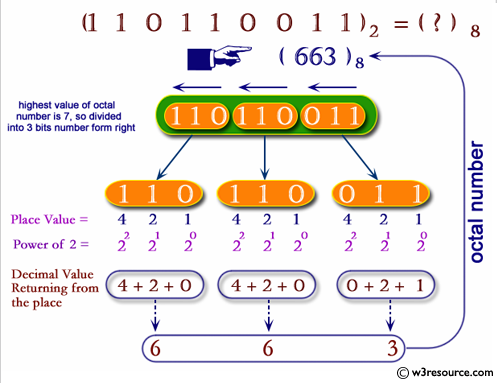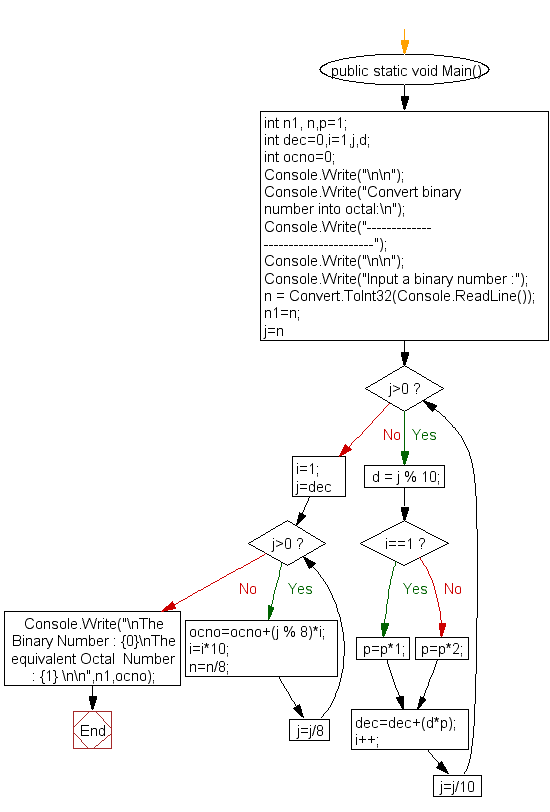﻿ C# - Convert binary number to octal - w3resource# C# Sharp Exercises: Convert binary number to octal

## C# Sharp For Loop: Exercise-53 with Solution

Write a program in C# Sharp to convert a binary number to octal.Sample Solution:-

C# Sharp Code:

``````using System;
public class Exercise53
{
public static void Main()
{       int n1, n,p=1;
int dec=0,i=1,j,d;
int ocno=0;

Console.Write("\n\n");
Console.Write("Convert binary number into octal:\n");
Console.Write("-----------------------------------");
Console.Write("\n\n");

Console.Write("Input a binary number :");
n1=n;
for (j=n;j>0;j=j/10)
{
d = j % 10;
if(i==1)
p=p*1;
else
p=p*2;

dec=dec+(d*p);
i++;
}

/*--------------------------------------------*/
i=1;

for(j=dec;j>0;j=j/8)
{
ocno=ocno+(j % 8)*i;
i=i*10;
n=n/8;
}

Console.Write("\nThe Binary Number : {0}\nThe equivalent Octal  Number : {1} \n\n",n1,ocno);
}
}
```
```

Sample Output:

```Convert binary number into octal:
-----------------------------------
Input a binary number :110101
The Binary Number : 110101
The equivalent Octal  Number : 65
```

Flowchart:C# Sharp Code Editor:

Contribute your code and comments through Disqus.

What is the difficulty level of this exercise?

Test your Programming skills with w3resource's quiz.

﻿